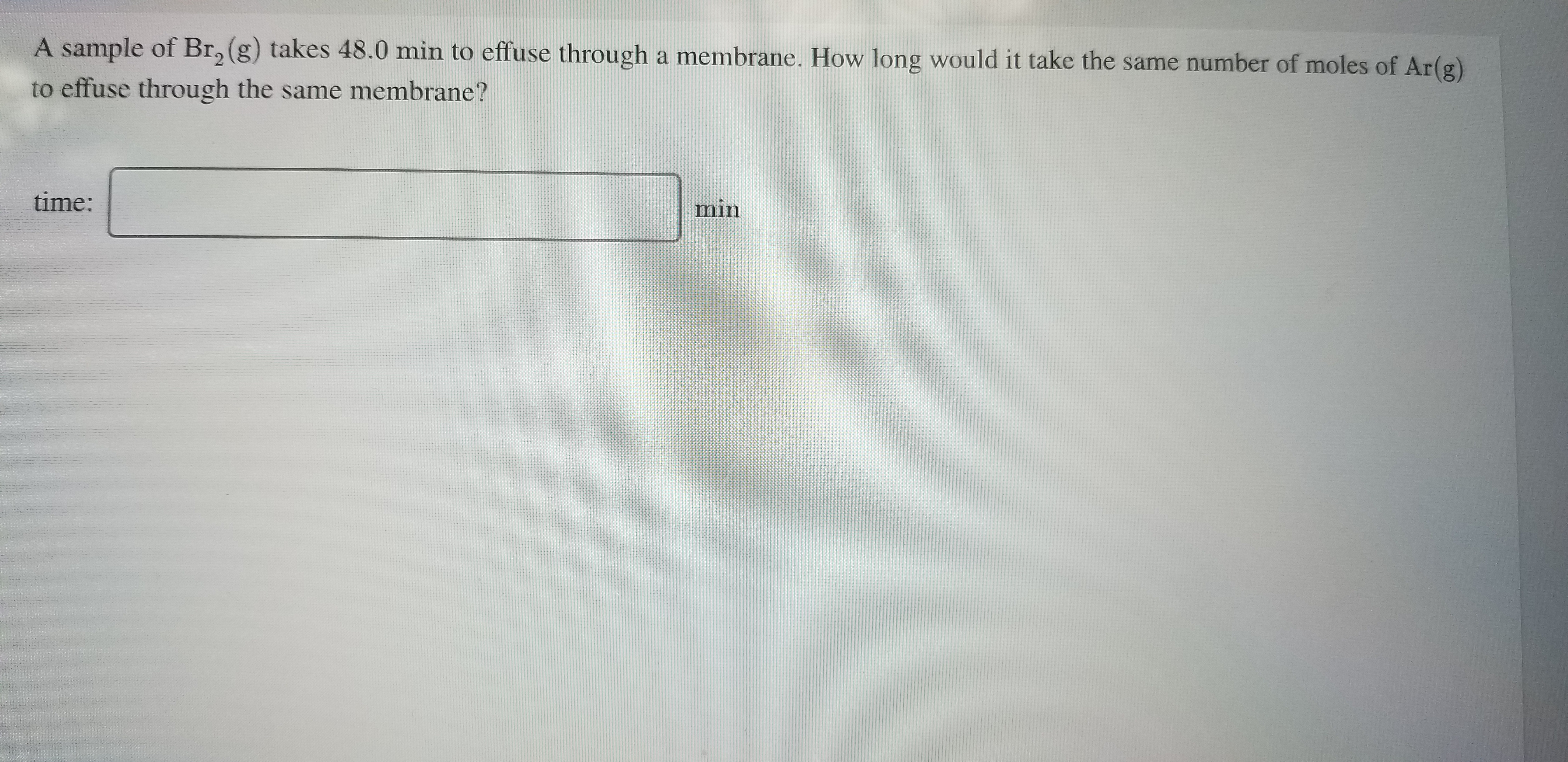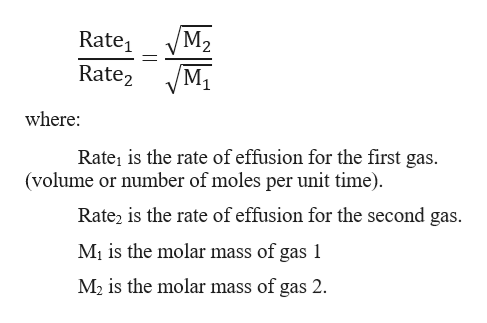# A sample of Br, (g) takes 48.0 min to effuse through a membrane. How long would it take the same number of moles of Ar(g)to effuse through the same membrane?time:min

Question
1 viewshelp_outlineImage TranscriptioncloseA sample of Br, (g) takes 48.0 min to effuse through a membrane. How long would it take the same number of moles of Ar(g) to effuse through the same membrane? time: min fullscreen
check_circle

Step 1

Graham`s law of diffusion: the rate of effusion of a gas is inversely proportional to the square root of the molar mass of its particles.

Step 2

For two gasses:help_outlineImage TranscriptioncloseM2 Rate, Rate2 VM1 where: Rate, is the rate of effusion for the first gas. (volume or number of moles per unit time). Rate, is the rate of effusion for the second gas. M¡ is the molar mass of gas 1 M, is the molar mass of gas 2. fullscreen
Step 3

Given:

Time of effusion of Br2 gas = 48 minutes

Molar mass of Br2 = 159.808...

### Want to see the full answer?

See Solution

#### Want to see this answer and more?

Solutions are written by subject experts who are available 24/7. Questions are typically answered within 1 hour.*

See Solution
*Response times may vary by subject and question.
Tagged in

### Chemistry